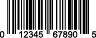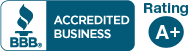# Check Digit Calculator

5-step algorithm for check digit calculation:

Let's assume that we are using the fictitious code 05432122345.
1. Add all of the digits in the odd positions (digits in position 1, 3, 5, 7, 9, and 11)
0 + 4 + 2 + 2 + 3 + 5 = 16
2. Multiply by 3.
16 * 3 = 48
3. Add all of the digits in even positions (digits in position 2, 4, 6, 8 and 10).
5 + 3 + 1 + 2 + 4 = 15
4. Sum the results of steps 3 and 2.
48 + 15 = 63
5. Determine what number needs to be added to the result of step 4 in order to create a multiple of 10.
63 + 7 = 70
The check digit therefore equals 7.

UPC bar code check digit calculator
Enter or paste 11 digit UPCDo you need professional print-ready digital UPC barcode graphics created? Please click here.

EAN bar code check digit calculator
Enter or paste 12 digit codeDo you need professional print-ready digital EAN barcode graphics created? Please click here.

If you need assistance creating check digits in bulk (from a database or an Excel file, for example) we can help! We can create check digits for just about any type of code (UPC, EAN, SCC-14/GTIN-14, SSCC-18, and many more).